# 978-0073382395 Chapter 10 Questions and Problems 1-15

Document Type
Homework Help
Book Title
Fundamentals of Corporate Finance Standard Edition 9th Edition
Authors
Stephen Ross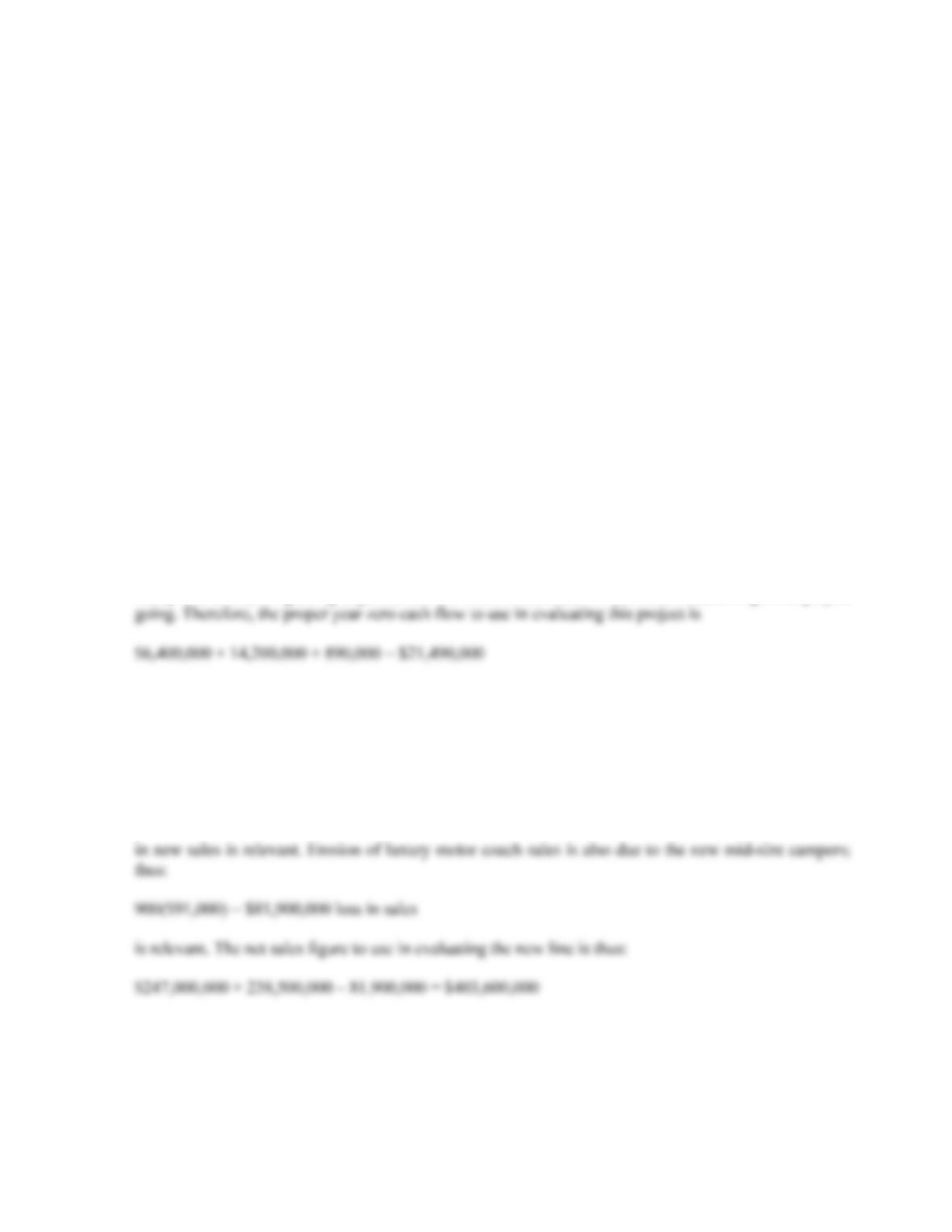B-178 SOLUTIONS
from the sale of new books. Thus, it is important to examine whether the new book would displace sales
of used books (good from the publisher’s perspective) or new books (not good). The concern arises any
time there is an active market for used product.
8. Definitely. The damage to Porsche’s reputation is definitely a factor the company needed to consider. If
the reputation was damaged, the company would have lost sales of its existing car lines.
9. One company may be able to produce at lower incremental cost or market better. Also, of course, one of
the two may have made a mistake!
10. Porsche would recognize that the outsized profits would dwindle as more product comes to market and
competition becomes more intense.
Solutions to Questions and Problems
NOTE: All end of chapter problems were solved using a spreadsheet. Many problems require multiple steps.
Due to space and readability constraints, when these intermediate steps are included in this solutions
manual, rounding may appear to have occurred. However, the final answer for each problem is found
without rounding during any step in the problem.
Basic
1. The \$6 million acquisition cost of the land six years ago is a sunk cost. The \$6.4 million current aftertax
value of the land is an opportunity cost if the land is used rather than sold off. The \$14.2 million cash
outlay and \$890,000 grading expenses are the initial fixed asset investments needed to get the project
2. Sales due solely to the new product line are:
19,000(\$13,000) = \$247,000,000
Increased sales of the motor home line occur because of the new product line introduction; thus:
4,500(\$53,000) = \$238,500,000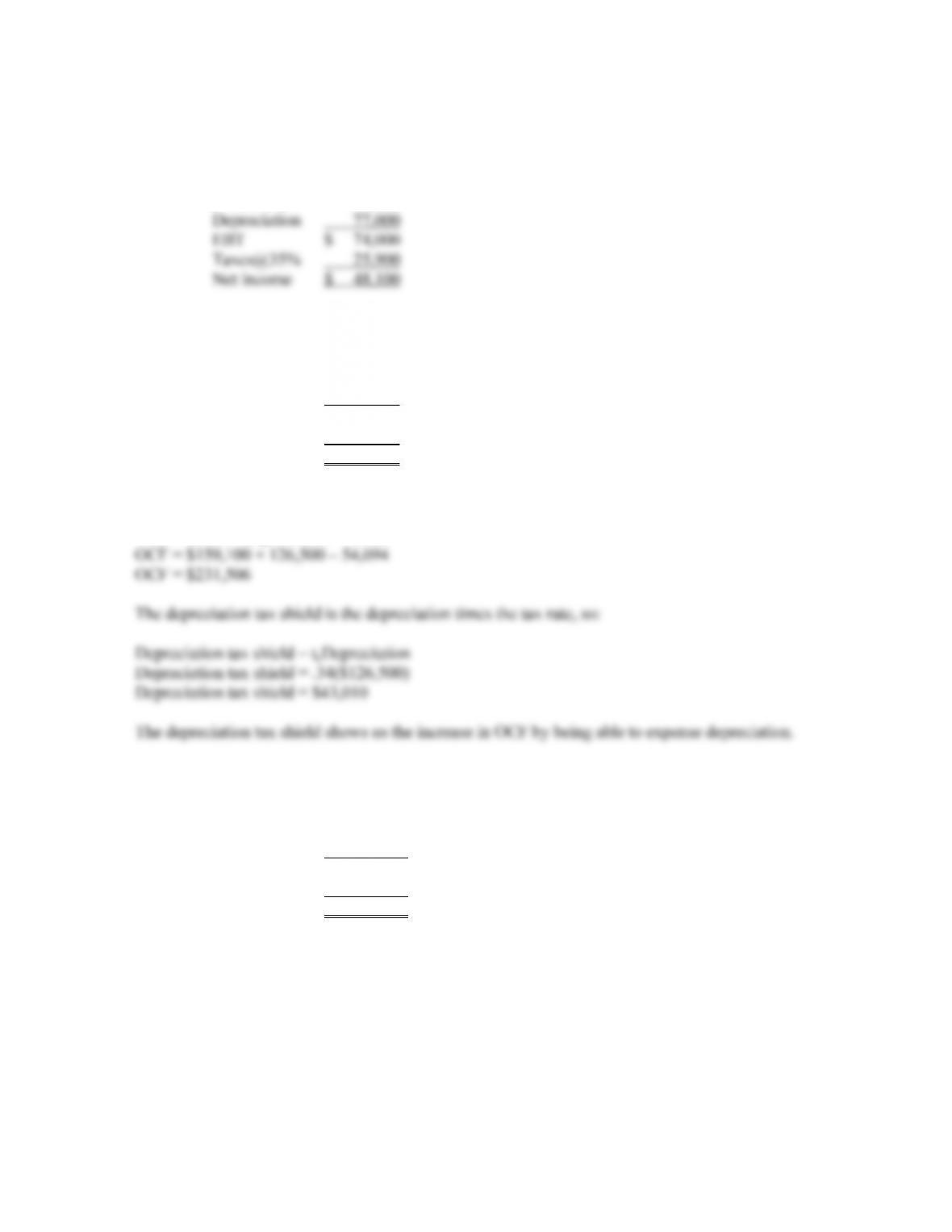CHAPTER 10 B-179
3. We need to construct a basic income statement. The income statement is:
Sales \$ 830,000
Variable costs 498,000
Fixed costs 181,000
4. To find the OCF, we need to complete the income statement as follows:
Sales \$ 824,500
Costs 538,900
Depreciation 126,500
EBT \$ 159,100
Net income \$ 105,006
The OCF for the company is:
OCF = EBIT + Depreciation – Taxes
5. To calculate the OCF, we first need to calculate net income. The income statement is:
Sales \$ 108,000
Variable costs 51,000
Depreciation 6,800
EBT \$ 50,200
Net income \$ 32,630
Using the most common financial calculation for OCF, we get:
OCF = EBIT + Depreciation – Taxes
OCF = \$50,200 + 6,800 – 17,570
OCF = \$39,430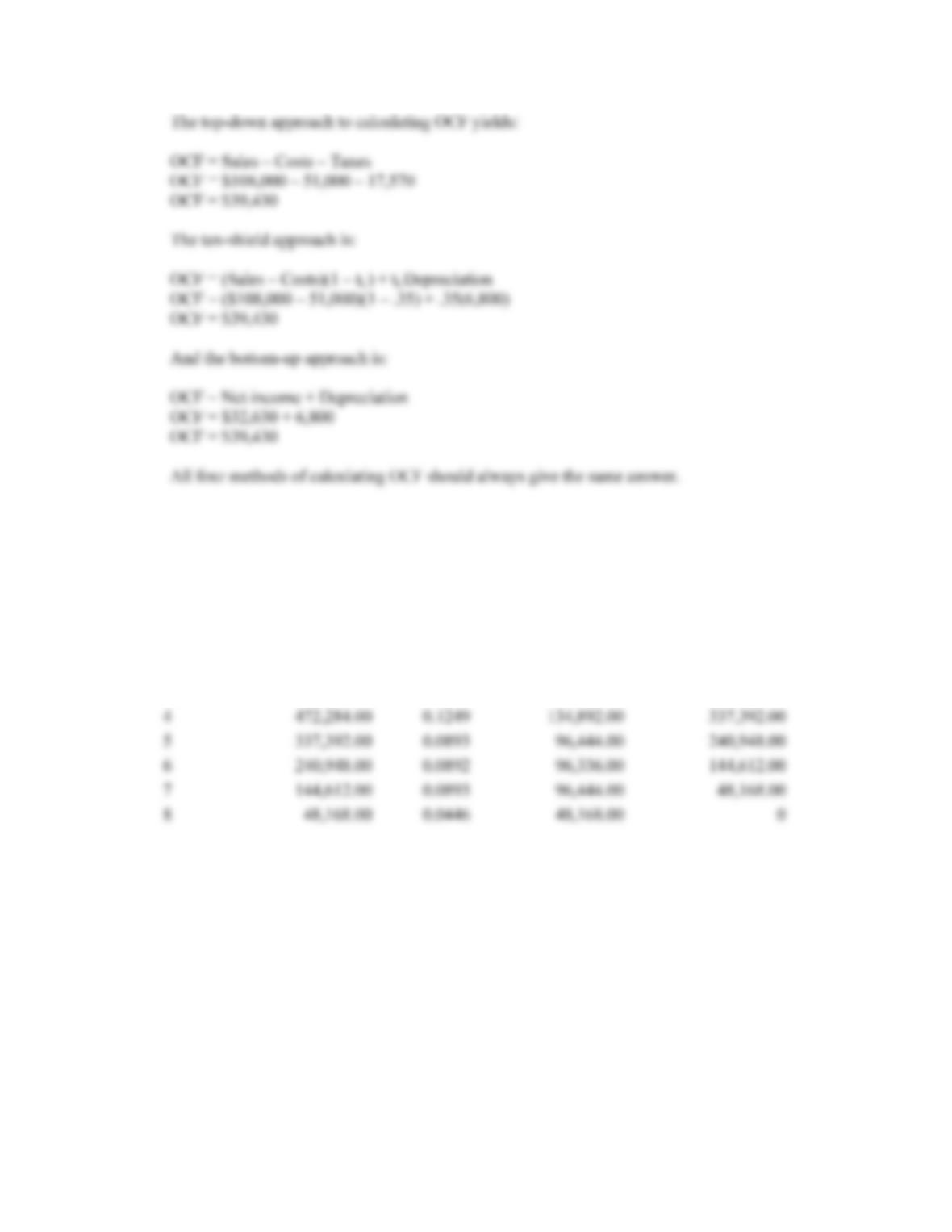B-180 SOLUTIONS
6. The MACRS depreciation schedule is shown in Table 10.7. The ending book value for any year is the
beginning book value minus the depreciation for the year. Remember, to find the amount of
depreciation for any year, you multiply the purchase price of the asset times the MACRS percentage for
the year. The depreciation schedule for this asset is:
Year Beginning Book Value MACRS Depreciation Ending Book value
1 \$1,080,000.00 0.1429 \$154,332.00 \$925,668.00
2 925,668.00 0.2449 264,492.00 661,176.00
3 661,176.00 0.1749 188,892.00 472,284.00
7. The asset has an 8 year useful life and we want to find the BV of the asset after 5 years. With straight-
line depreciation, the depreciation each year will be:
Annual depreciation = \$548,000 / 8
Annual depreciation = \$68,500
So, after five years, the accumulated depreciation will be:
Accumulated depreciation = 5(\$68,500)
Accumulated depreciation = \$342,500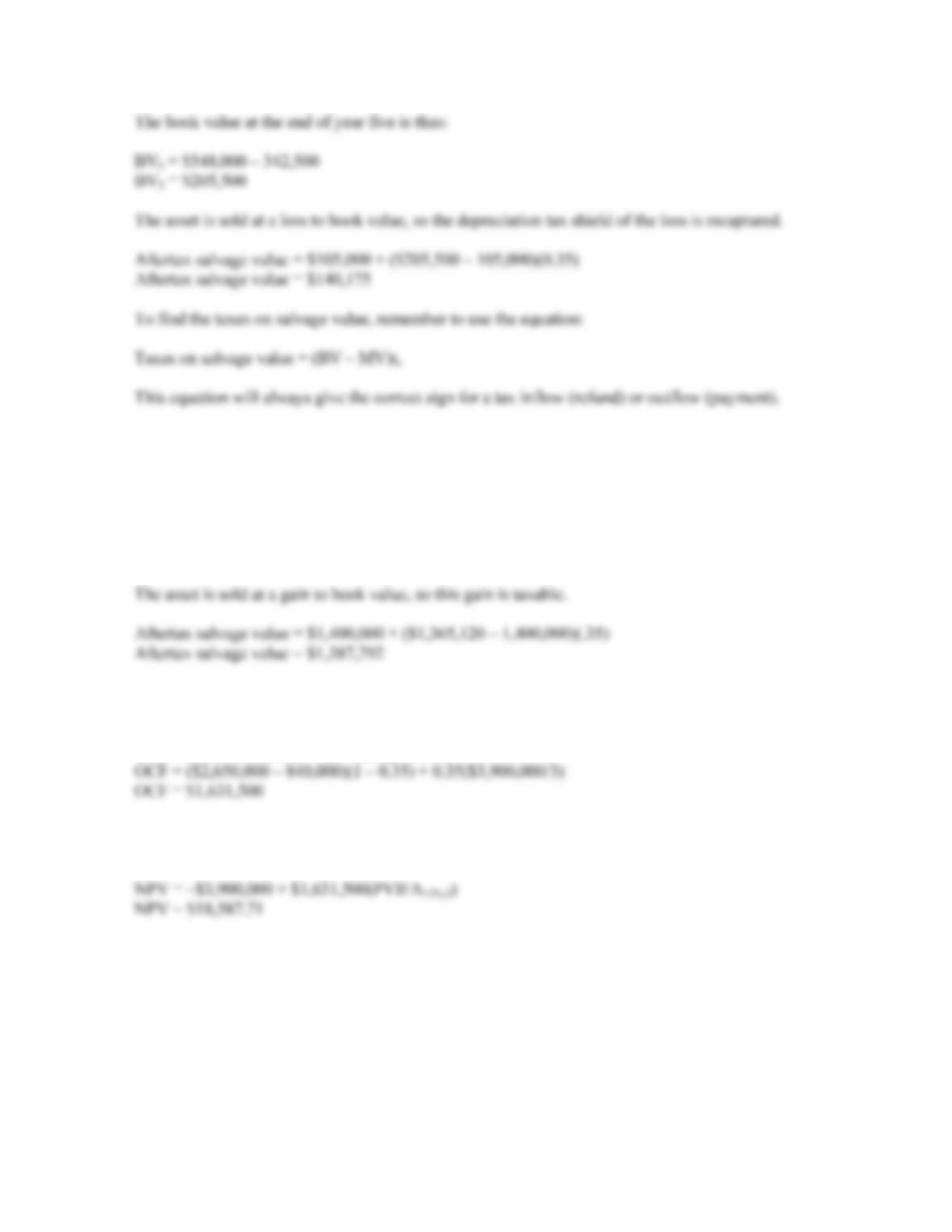CHAPTER 10 B-181
8. To find the BV at the end of four years, we need to find the accumulated depreciation for the first four
years. We could calculate a table as in Problem 6, but an easier way is to add the MACRS depreciation
amounts for each of the first four years and multiply this percentage times the cost of the asset. We can
then subtract this from the asset cost. Doing so, we get:
BV4 = \$7,900,000 – 7,900,000(0.2000 + 0.3200 + 0.1920 + 0.1152)
BV4 = \$1,365,120
9. Using the tax shield approach to calculating OCF (Remember the approach is irrelevant; the final
answer will be the same no matter which of the four methods you use.), we get:
OCF = (Sales – Costs)(1 – tC) + tCDepreciation
10. Since we have the OCF, we can find the NPV as the initial cash outlay plus the PV of the OCFs, which
are an annuity, so the NPV is: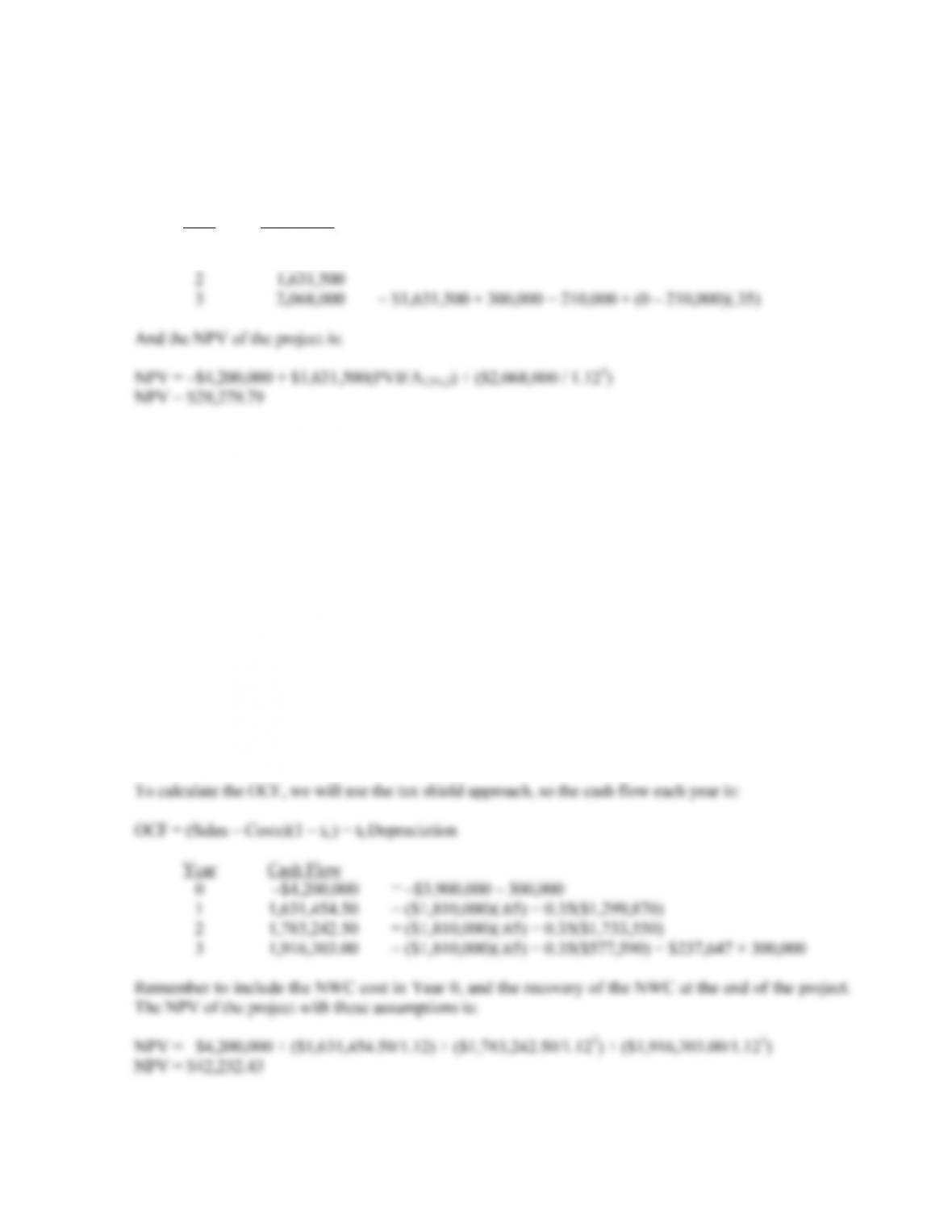B-182 SOLUTIONS
11. The cash outflow at the beginning of the project will increase because of the spending on NWC. At the
end of the project, the company will recover the NWC, so it will be a cash inflow. The sale of the
equipment will result in a cash inflow, but we also must account for the taxes which will be paid on this
sale. So, the cash flows for each year of the project will be:
Year Cash Flow
0 –\$4,200,000 = –\$3,900,000 – 300,000
1 1,631,500
12. First we will calculate the annual depreciation for the equipment necessary for the project. The
depreciation amount each year will be:
Year 1 depreciation = \$3,900,000(0.3333) = \$1,299,870
Year 2 depreciation = \$3,900,000(0.4445) = \$1,733,550
Year 3 depreciation = \$3,900,000(0.1481) = \$577,590
So, the book value of the equipment at the end of three years, which will be the initial investment minus
the accumulated depreciation, is:
Book value in 3 years = \$3,900,000 – (\$1,299,870 + 1,733,550 + 577,590)
Book value in 3 years = \$288,990
The asset is sold at a loss to book value, so this loss is taxable deductible.
Aftertax salvage value = \$210,000 + (\$288,990 – 210,000)(0.35)
Aftertax salvage value = \$237,647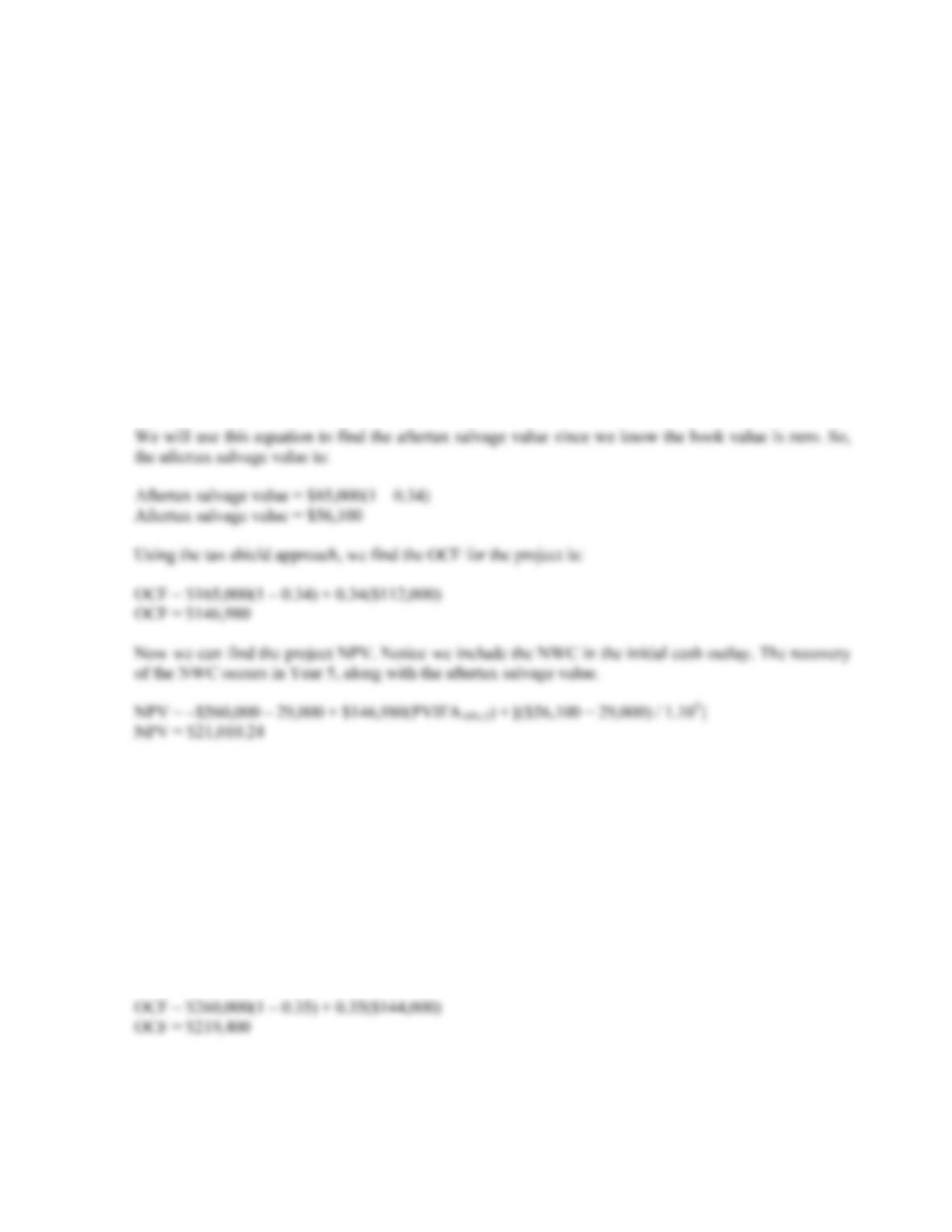CHAPTER 10 B-183
13. First we will calculate the annual depreciation of the new equipment. It will be:
Annual depreciation = \$560,000/5
Annual depreciation = \$112,000
Now, we calculate the aftertax salvage value. The aftertax salvage value is the market price minus (or
plus) the taxes on the sale of the equipment, so:
Aftertax salvage value = MV + (BV – MV)tc
Very often the book value of the equipment is zero as it is in this case. If the book value is zero, the
equation for the aftertax salvage value becomes:
Aftertax salvage value = MV + (0 – MV)tc
Aftertax salvage value = MV(1 – tc)
14. First we will calculate the annual depreciation of the new equipment. It will be:
Annual depreciation charge = \$720,000/5
Annual depreciation charge = \$144,000
The aftertax salvage value of the equipment is:
Aftertax salvage value = \$75,000(1 – 0.35)
Aftertax salvage value = \$48,750
Using the tax shield approach, the OCF is: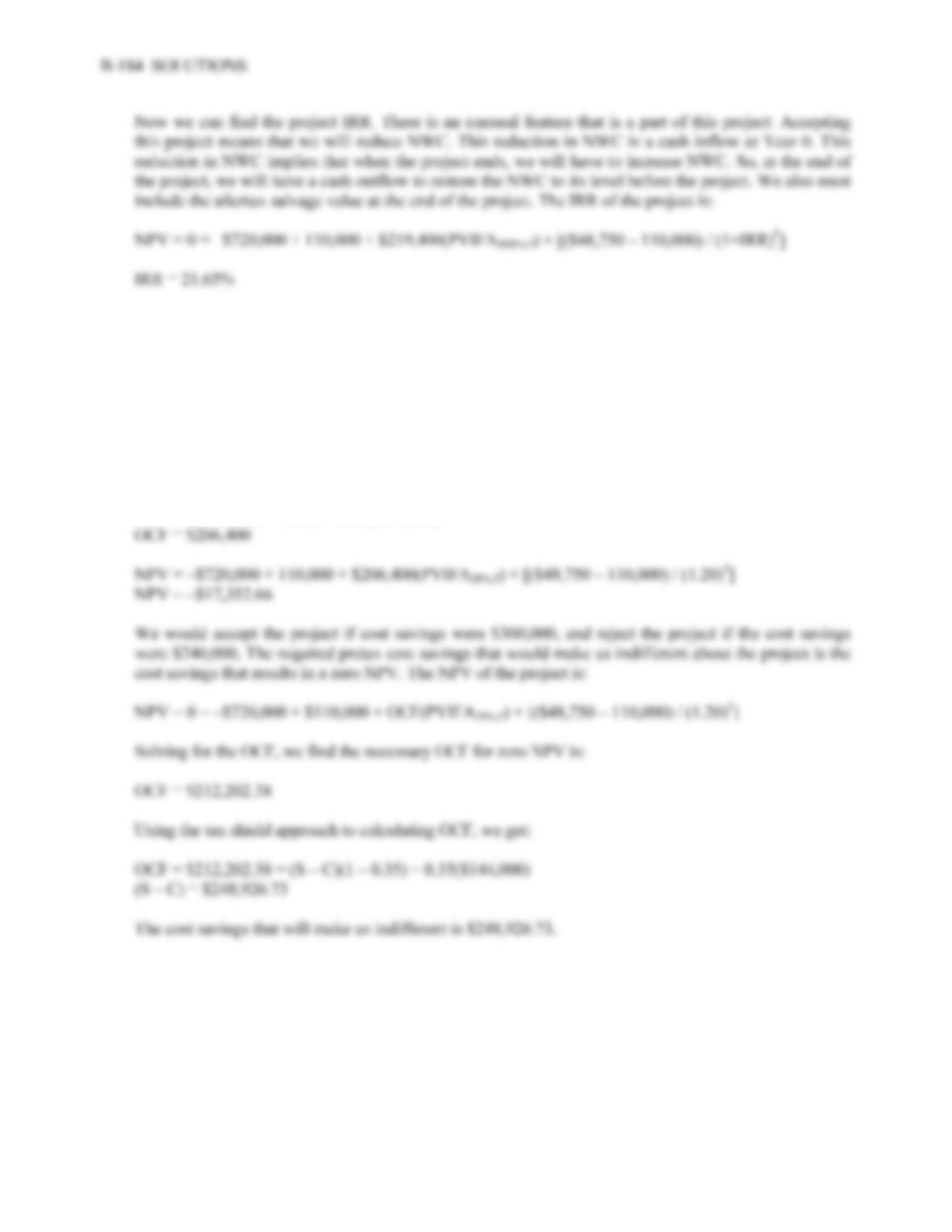15. To evaluate the project with a \$300,000 cost savings, we need the OCF to compute the NPV. Using the
tax shield approach, the OCF is:
OCF = \$300,000(1 – 0.35) + 0.35(\$144,000) = \$245,400
NPV = –\$720,000 + 110,000 + \$245,400(PVIFA20%,5) + [(\$48,750 – 110,000) / (1.20)5]
NPV = \$99,281.22
The NPV with a \$240,000 cost savings is:
OCF = \$240,000(1 – 0.35) + 0.35(\$144,000)

## Trusted by Thousands ofStudents

Here are what students say about us.